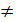Daily Practice Problems
Class 7 Maths
IntegersQuestion 1:

1. The sum of two integers is -6. If one of them is 2, then the other is

(a) -4

(b) 4

(c) 8

(d) -8

2. What should be subtracted from -7 to obtain -15

(a) -8

(b) 8

(c) -22

(d) 22

3. (?) ÷ (-18) = - 6

(a) -108

(b) 108

(c) 3

(d) None of these

4. ( - 37)  X ( - 7) + ( - 37) X ( - 3) = ?

(a) 370

(b) -370

(c) 148

(d) -148

5. (-9) – (-6) =?

(a) -15

(b) -3

(c) 3

(d) 15

Question 2:

Find the value of:

(i) 1487 × 327 + (-487) × 327

(ii) 28945 × 99 – (-28945)

Question 3:

A shopkeeper gains Rs 1 on each pen and loses 40 paise on each pencil. He sells 45 pens and some pencil losing Rs 5 in all. How many pencils does he sell?

Question 4:

Solve the following:

(a) (-40) × (-1) + (-28) ÷ 7

(b) (-3) + (-8) ÷ (-4) -2 × (-2)

Question 5:

Subtract -134 from the sum of 38 and -87

Question 6:

A certain freezing process requires that room temperature we lowered from 400C at the rate of 50C per hour. What will be the room temperature 12 hours after the process begins?

Question 7:

In a class test containing 20 questions, 4 marks are given for every correct answer and (-2) marks are given for every incorrect answer. Ranjita attempts all questions and 12 of her answers are correct. What is her total score?

Question 8:

Add the product of (-16) and (-9) to the quotient of (-132) by 6.

Question 9:

Divide:

(i) (-115) by 23

(ii) (-324) by (- 27)

Question 10:

What should be divided by (- 7) to obtain 12?

Question 11:

What will be the sign of the product if we multiply together 199 negative and 10 positive integers?

Question 12:

Find each of the following products:

(i) 35 X (-18)

(ii) (-42) X 20

Question 13:

Simplify:

(i) 8 X (-15) + 8 X 6

(ii) 15 X (-32) + 15 X (-18)

Question 14:

Find 36 – (-64) and (-64 )- 36 . Are they equal?

Question 15:

If a = -9 and b = -6, show that (a – b)(b – a)

Question 16:

In a class test containing 10 questions, 5 marks are awarded for correct answer and (-2) marks are awarded for every incorrect answer and 0 for each question not attempted. Ravi gets 4 correct and 6 incorrect answers. What is his score?

Question 17:

Fill in the blanks:

(i) (- 6) X (___) = 6

(ii) (-18) X (___) = -18

(iii) (-8) X (-9) = (- 9) X (___)

Question 18:

For each of the following statements, write (T) for True and (F) for false:

(i) The smallest integer is zero

(ii) -10 is greater than -7

(iii) Zero is larger than every negative integer.

Question 19:

Subtract -134 from the sum of 38 and -87.

Question 20:

Find each of the following products:

(i) (-4) X (- 5) X (-8) x (-10)

(ii) 7 x (-8) X 3

**********